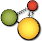# CindyJS## Elementary List Operations

### Creating and Accessing Lists

#### Creating an integer sequence: `‹int1›..‹int2›`

Description: The expression `‹int1›..‹int2›` creates a list of consecutive integers starting with `‹int1›` and ending with `‹int2›`. If `‹int1›` is larger than `‹int2›`, then the empty list is returned.

`4..9`
`[4, 5, 6, 7, 8, 9]`
`-2..2`
`[-2, -1, 0, 1, 2]`
`4..1`
`[]`

#### The length of a list: `length(‹list›)`

Description: This operator returns an integer that is equal to the number of elements in the `‹list›`.

`length([2 ,5 ,7 ,3])`
`4`
`length([2 ,[5, 4, 5] ,7 ,3]_2)`
`3`
`length(1..1000)`
`1000`

Combining the `length` and the `repeat` operator allows one to list all elements of a list easily.

```list = [2,3,5,7];
repeat(length(list),
println(list_#);
)```
```2
3
5
7```

One word of caution here: CindyScript is designed in such a way that it is seldom useful to traverse all the elements of a list using the `repeat` operator. There are more elegant ways.

#### Testing for containment: `contains(‹list›,‹expr›)`

Description: This operator returns either `true` or `false` depending on whether `‹list›` contains the element `‹expr›`.

`contains([1,3,4,5],4)`
`true`
`contains([1,3,4,5],7)`
`false`
`contains([1,3,4,5],2*2)`
`true`
`CindyScript >=3.0`
`4 ∈ [1,3,4,5]`
`true`
`7 ∈ [1,3,4,5]`
`false`
`4 ∉ [1,3,4,5]`
`false`
`7 ∉ [1,3,4,5]`
`true`

### List Manipulation

#### Concatenation of lists: `‹list1› ++ ‹list2›`, `‹list1› ∪ ‹list2›` or `concat(‹list1›,‹list2›)`

Description: This operator creates a list by concatenation of two other lists.

`concat(["a", "b"], ["c", "d"])`
`["a", "b", "c", "d"]`

Thanks to auto-coercion, numbers and boolean values will get converted to singleton lists.

`concat(2, true)`
`[2, true]`

Strings and `___` auto-coerce to the empty list.

`concat("foo", )`
``
`concat(, (;))`
``

#### Removing elements from lists: `‹list1› -- ‹list2›`, `‹list1› ∖ ‹list2›` or `remove(‹list1›,‹list2›)`

Description: This operator creates a list by removing all elements that occur in `‹list2›` from `‹list1›`. Note that `∖` is not a plain backslash, but the Unicode symbol ‘set minus’.

`remove([1,3,4,5,1,5,6], [1,3,7])`
`[4, 5, 5, 6]`
`[1,3,4,5,1,5,6]--[1,3,7]`
`[4, 5, 5, 6]`

#### Intersection of lists: `‹list1› ~~ ‹list2›`, `‹list1› ∩ ‹list2›` or `common(‹list1›,‹list2›)`

Description: This operator creates a list collecting all elements that are in both `‹list1›` and `‹list1›`. In the returned list the elements are sorted and each element occurs at most once.

`common([1,3,4,5,1,5,6], [1,3,7])`
`[1, 3]`
`[1,3,4,5,1,5,6]~~[1,3,7]`
`[1, 3]`

#### Appending an element: `‹list› :> ‹expr›` or `append(‹list›,‹expr›)`

Description: This operator returns a list that is created by appending `‹expr›` to the list `‹list›` as its last element.

`append(["a", "b", "c"], "d")`
`["a", "b", "c", "d"]`
`["a", "b", "c"]:>"d"`
`["a", "b", "c", "d"]`

#### Prepending an element: `‹expr› <: ‹list›` or `prepend(‹expr›,‹list›)`

Description: This operator returns a list that is created by prepending `‹expr›` to the list `‹list›` as its first element.

`prepend("d",["a", "b", "c"])`
`["d", "a", "b", "c"]`
`"d"<:["a", "b", "c"]`
`["d", "a", "b", "c"]`

### Traversing Lists

#### The forall loop: `forall(‹list›,‹expr›)`

Description: This operator is useful for applying an operation to all elements of a list. It takes a `‹list›` as first argument. It produces a loop in which `‹expr›` is evaluated for each entry of the list. For each run, the run variable `#` takes the value of the corresponding list entry.

Example:

```a=["this","is","a","list"];
forall(a,println(#))```

This code fragment produces the output

```this
is
a
list```

#### The forall loop: `forall(‹list›,‹var›,‹expr›)`

Description: Similar to `forall(‹list›,‹expr›)`, but the run variable is now named `‹var›`. The variable is local to the expression.

```v=994;
forall([1,2,3],v,println(v))```
```1
2
3```
`v`
`994`

#### Applying an expression: `apply(‹list›,‹expr›)`

Description: This operator generates a new list by applying the operation `‹expr›` to all elements of a list and collecting the results. As usual, `#` is the run variable, which successively takes the value of each element in the list.

`apply([1, 2, 3, 4, 5],#^2)`
`[1, 4, 9, 16, 25]`
`apply([1, 2, 3, 4, 5],#+5)`
`[6, 7, 8, 9, 10]`
`apply(1..5, [#,#^2])`
`[[1, 1], [2, 4], [3, 9], [4, 16], [5, 25]]`

#### Applying an expression: `apply(‹list›,‹var›,‹expr›)`

Description: Similar to `apply(‹list›,‹expr›)`, but the run variable is now named `‹var›`. The variable is local to the expression.

```v=995;
apply([1, 2, 3, 4, 5], v, v^2)```
`[1, 4, 9, 16, 25]`
`v`
`995`

#### Selecting elements of a list: `select(‹list›,‹boolexpr›)`

Description: This operator selects all elements of a list for which a certain condition is satisfied. The condition is supposed to be encoded by `‹boolexpr›`. This expression is assumed to return a `‹bool›` value. As usual, `#` is the run variable, which successively take the value of all elements in the list.

`select(1..10, isodd(#))`
`[1, 3, 5, 7, 9]`
`select(0..10, #+# == #^2)`
`[0, 2]`

A high-level application of the `select` operator is given by the following example:

```divisors(x):=select(1..x,mod(x,#)==0);
primes(n):=select(1..n,length(divisors(#))==2);
println(primes(20))```

It produces the output

`[2, 3, 5, 7, 11, 13, 17, 19]`

In this example, first a function `divisors(x)` is defined by selecting those numbers that divide `x` without any remainder. Then a function `primes(n)` is defined that selects all numbers between `1` and `n` that have exactly two divisors. These numbers are the primes.

#### Selecting elements of a list: `select(‹list›,‹var›,‹boolexpr›)`

Description: Similar to `select(‹list›,‹boolexpr›)`, but the run variable is now named ‹var›. The variable is local to the expression.

```v = 996;
select(0..10, v, v+v == v^2)```
`[0, 2]`
`v`
`996`